## 4.1.10 Code search algorithm

06.203GPPHalf rate speech transcodingTS

For MODE¹0, the excitation codebook search procedure takes place after the long term predictor lag, L, has been determined. The codebook search procedure then chooses one codevector from the VSELP codebook. The GSM half rate speech encoder uses an excitation codebook of 2M codevectors which is constructed from M basis vectors. Defining vm(n) as the mth basis vector and ui(n) as the ith codevector in the codebook, then: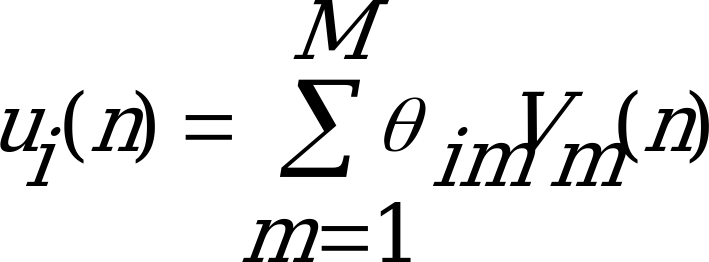(108)

where 0 £ i £ 2M‑1; 0 £ n £ Ns‑1. In other words, each codevector in the codebook is constructed as a linear combination of the M basis vectors. The linear combinations are defined by the  parameters.

im is defined as:

im = + 1 if bit m of codeword i = 1

im = – 1 if bit m of codeword i = 0

The codebook construction for the GSM half rate speech encoder can be restated as follows. Codevector i is constructed as the sum of the M basis vectors where the sign (plus or minus) of each basis vector is determined by the state of the corresponding bit in codeword i. The codebook search procedure finds the codevector which will produce the minimum total spectral and harmonic weighted error for the subframe given b"L(n) (the zero state response of H(z)C(z) to bL(n) ) and allowing both the gain, , and the long term filter coefficient, , to be optimized for each codevector being evaluated.

The filtered codevector fi(n), can be expressed as: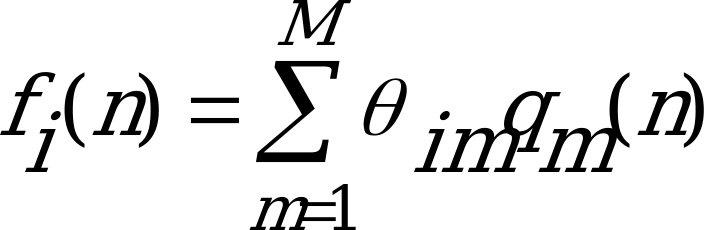(109)

where qm(n) is the zero state response of H(z)C(z) to basis vector vm(n).

If MODE=0, two VSELP codebooks are the excitation sources and are searched sequentially to identify the codeword I specifying the codevector selected from the first codebook, and codeword H, identifying the codevector chosen from the second VSELP codebook. Harmonic noise weighting is not used. When searching the second VSELP codebook, each codevector is evaluated assuming optimal gains for the codevector I, and the potential codevector H.

#### 4.1.10.1 Decorrelation of filtered basis vectors

For MODE¹0, each filtered codevector, fi(n), is decorrelated to the long term predictor vector, b"L(n). If MODE=0, the bL(n) vector and the single VSELP codebook excitation are replaced by two VSELP codebook excitations, as the excitation sources. In this case, decorrelation is only performed for the second VSELP codebook. In this case, the orthogonalization is done with respect to the filtered codevector chosen from the first VSELP codebook.

Defining: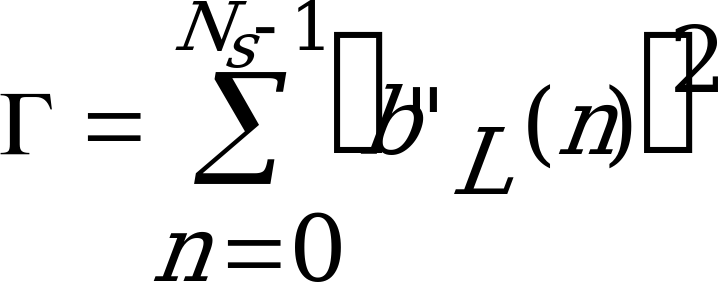(110)

and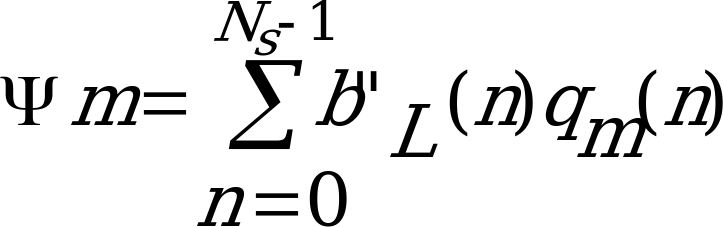(111)

for 1 £ m £ M; then q’m(n), the decorrelated filtered basis vectors, can be computed by: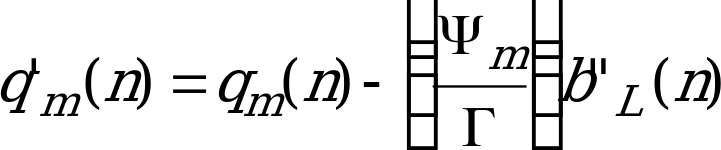(112)

for 1 £ m £ M and 0 £ n £ Ns‑1.

The decorrelated filtered codevectors can now be expressed as: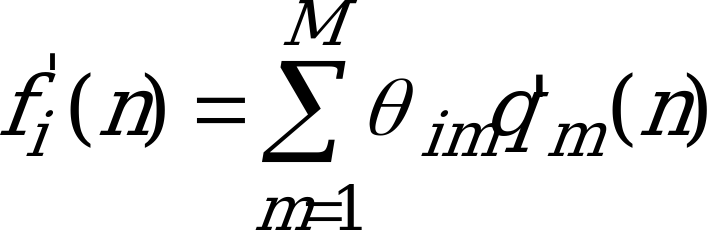(113)

for 0 £ i £ 2M‑1 and 0 £ n £ Ns‑1.

#### 4.1.10.2 Fast search technique

The codebook search procedure should find the codeword i which minimizes: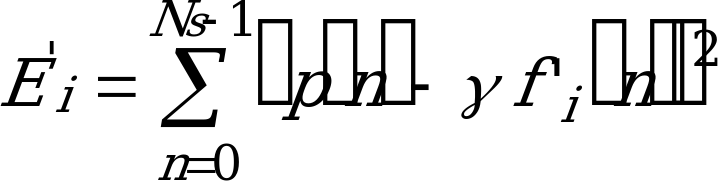(114)

Defining :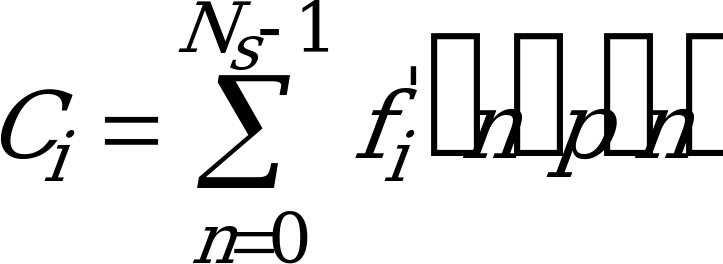(115)

and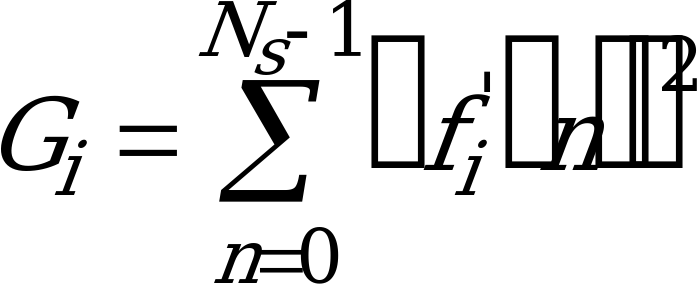(116)

then the best codevector is the one which maximizes: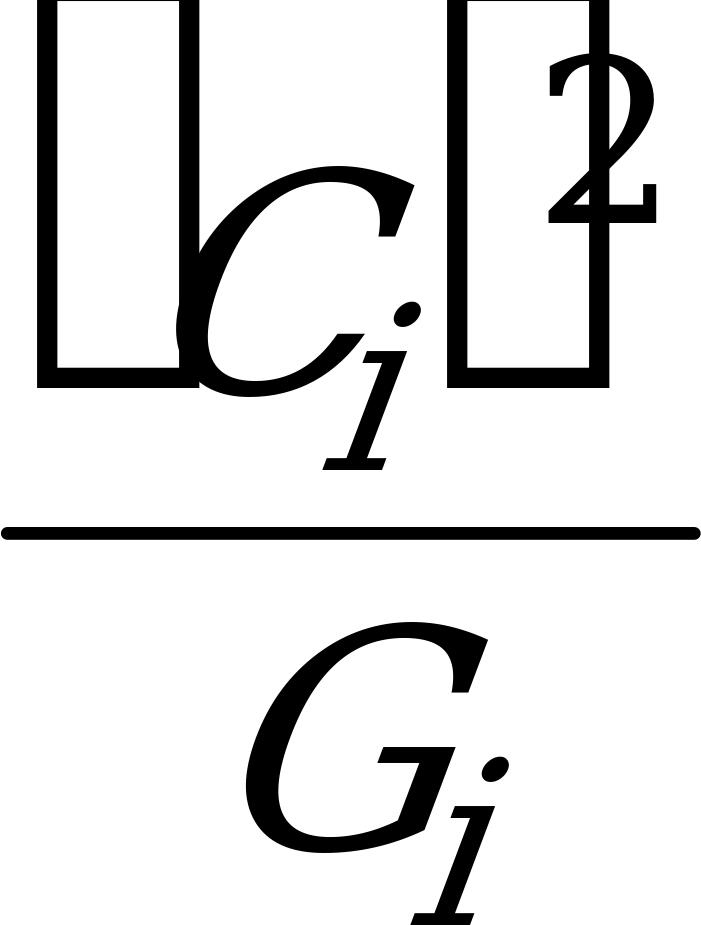(117)

and the corresponding optimal gain is given by: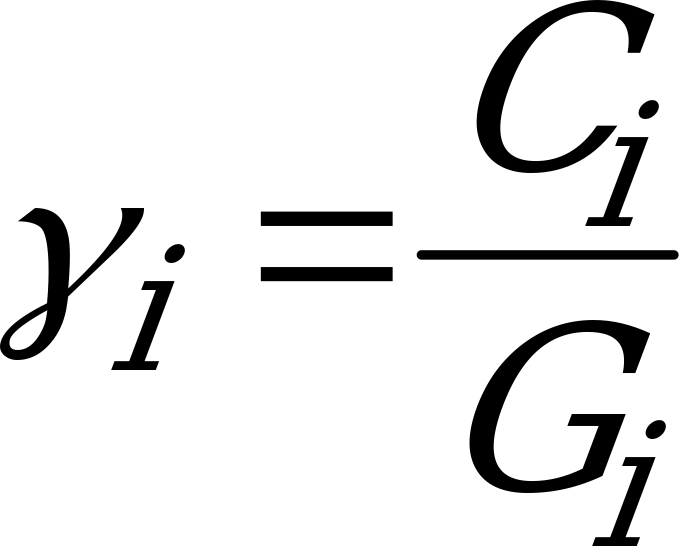(118)

The search process needs to evaluate equation (117) for each codevector. The codevector which maximizes equation (117) is then chosen. Using properties of the VSELP codebook construction, the computations required for computing Ci and Gi can be greatly simplified.

defining: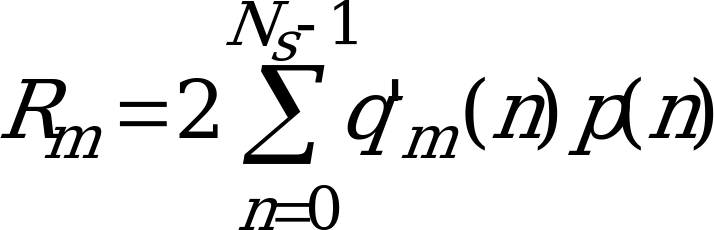(119)

for 1 £ m £ M and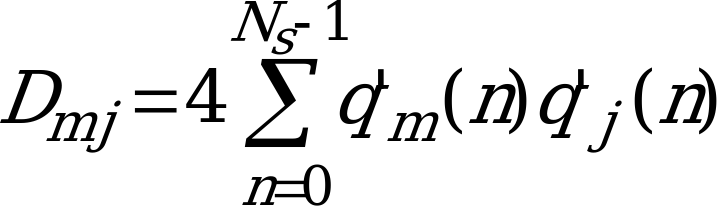(120)

for 1 £ m £ j £ M

Ci can be expressed as: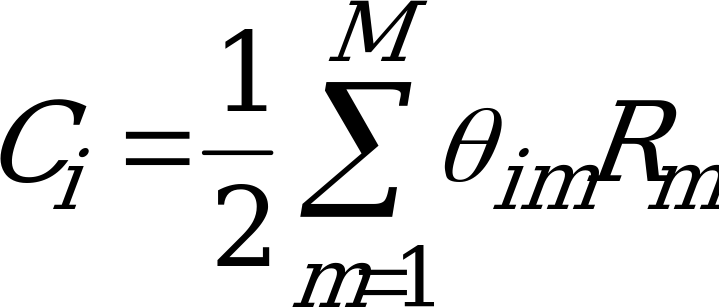(121)

and Gi can be expressed as: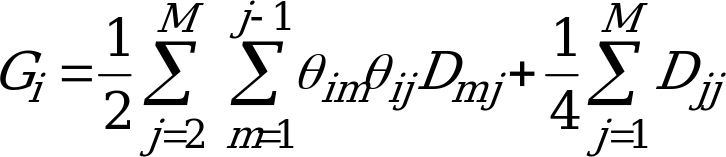(122)

Assuming that codeword u differs from codeword i in only one bit position, say position v such that
quv = ‑qiv and qum = qim for m ¹ v then:(123)

and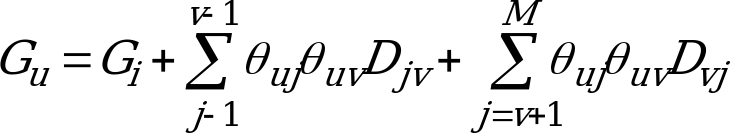(124)

The codebook search is structured such that each successive codeword evaluated differs from the previous codeword in only one bit position, then equation (123) and equation (124) can be used to update Ci and Gi in a very efficient manner. Sequencing of the codewords in this manner is accomplished using a binary Gray code. This updating operation for both equation (123) and equation (124) can be accomplished with a total of only M multiply-accumulates per codevector.

With this technique for computing Ci and Gi for the codevectors in a VSELP codebook, it is necessary to find the i which maximizes equation (117). Note that complementary codewords (see subclause 3.1.10) will have equivalent values for equation (117). Therefore only half of the codevectors need to be evaluated. Once the codevector which maximizes equation (117) is found, the sign of Ci for that codevector will determine whether that codevector or its complement will yield a positive gain, .

A running maximum for equation (117) is kept during the code search then for each codevector evaluated, evaluate equation (117) and compare to the running maximum.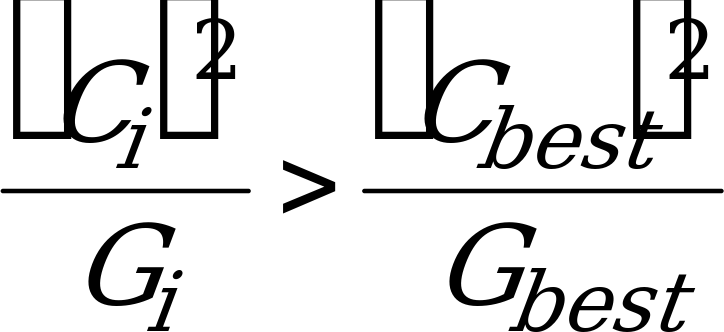(125)

Evaluating equation (125) directly from Ci and Gi requires one multiply, one divide and one compare operation. By cross multiplying equation (117) can be expressed as: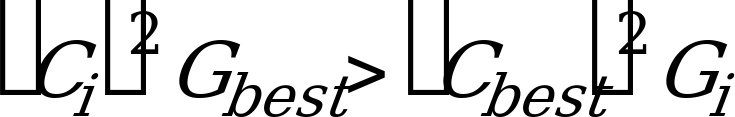(126)

Using equation (126) requires only three multiplies and a compare per evaluation (and no divides) where (Cbest)2 and Gbest are updated throughout the search to reflect the running best codeword.